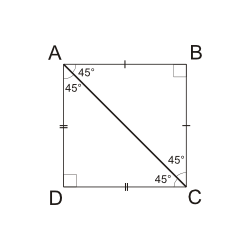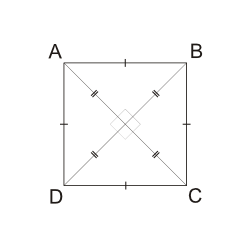We cover every section of the GMAT with in-depth lessons, 5000+ practice questions and realistic practice tests.

## Up to 90+ points GMAT score improvement guarantee

### The best guarantee you’ll find

Our Premium and Ultimate plans guarantee up to 90+ points score increase or your money back.

## Master each section of the test

### Comprehensive GMAT prep

We cover every section of the GMAT with in-depth lessons, 5000+ practice questions and realistic practice tests.

## Schedule-free studying

### Learn on the go

Study whenever and wherever you want with our iOS and Android mobile apps.

## The most effective way to study

### Personalized GMAT prep, just for you!

Is quadrilateral \$\$ABCD\$\$ a square? >(1) \$\$ABCD\$\$ is a rectangle whose diagonals are perpendicular. >(2) Triangles \$\$ABC\$\$ and \$\$ADC\$\$, formed by line segment \$\$AC\$\$, are isosceles triangles where angles \$\$ABC\$\$ and \$\$ADC\$\$ are right angles.
Incorrect. [[snippet]] Either one of the statements is sufficient on its own.
Stat. (2): Now try to draw quadrilateral \$\$ABCD\$\$ based on Stat.(2). Right isosceles triangles are 45º-45º-90º recycled right triangles. This means that the other two angles of \$\$ABCD\$\$ are also right angles (the sum of 45 and 45, as the figure below shows).As for the sides, we have two pairs of equal sides with the same hypotenuse \$\$AC\$\$. Using the Pythagorean theorem, we get \$\$AB^2+ AB^2 = AC^2 = CD^2 + CD^2\$\$ and therefore > \$\$AB=BC=CD=DA\$\$ So all angles in \$\$ABCD\$\$ are 90° and all sides are equal. That's a square! Stat.(2) → Yes → S → D.
Incorrect. [[snippet]] Either one of the statements is sufficient on its own.
Incorrect. [[snippet]] Stat.(2) is sufficient. But what about Stat.(1)?
Incorrect. [[snippet]] Stat.(1) is sufficient. But what about Stat.(2)?
Correct. [[snippet]] Stat. (1): \$\$ABCD\$\$'s diagonals are perpendicular to one another based on the statement, and they bisect one another since diagonals in a rectangle always bisect one another. This creates four right isosceles triangles within \$\$ABCD\$\$ (see figure below), in which both legs are half the diagonal's length. Based on the Pythagorean theorem, all of the hypotenuses should also be equal, so \$\$AB=BC=CD=DA\$\$. Combined with the four right angles of a rectangle, you get a square. Stat.(1) → Yes → S → AD.Statement (1) ALONE is sufficient, but Statement (2) alone is not sufficient to answer the question asked.
Statement (2) ALONE is sufficient, but Statement (1) alone is not sufficient to answer the question asked.
BOTH statements (1) and (2) TOGETHER are sufficient to answer the question asked, but NEITHER statement ALONE is sufficient to answer the question asked.